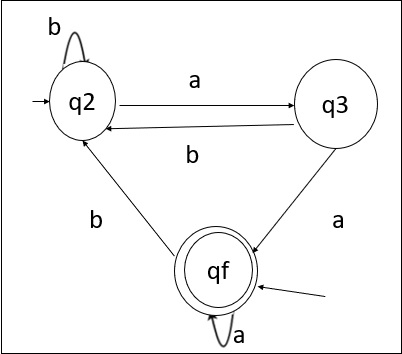# Explain Arden’s theorem to convert DFA to Regular Expression

There are two methods for converting a Deterministic Finite Automata (DFA) to Regular expression (RE). These methods are as follows −

• Arden's Theorem Method.
• State Elimination Method.

Let us understand the Arden's Theorem method in detail.

## Arden's Theorem

Let P and Q be the two regular expressions.

If P does not contain null string, then the following equation in R, viz R = Q + RP,

Which has a unique solution by R = QP*

Here,

• The finite automata (FA) does not have epsilon moves.
• It must have only initial state q1.
• Its states are q1, q2, q3,....qn. The final state may be some qi where i<=n.
• qi is the regular expression representing a set of strings accepted by the finite automata even though qi is a final state.

## Use of Arden's Theorem to find RE of DFA.

To find the regular expression for the given automata, we can first create the equations in the given form for all the states.

q1=q1α11+q2α21+----------+qnαn1+ε

q2=q1α12+q2α22+-----------+qnαn2

-

-

-

-

qn=q1α1n+q2α2n+------------+qnαnn.

By repeatedly applying substitutions and Arden's Theorem we can express qi in terms of α ij's. To get the set of strings recognized by the Finite State Automata (FSA), we have to take the union of all qi's corresponding to final states

## Example

Note: For the parallel edges, there will be many expressions for that state in the expression.

Then, we solve those equations to get the equation for qi in terms of α ij and that expression is the required solution, where qi is a final state. It is shown below −q 1= q a + q3a + € ( € move is because q1is the initial state)

q2 = q1b + q2b + q3b

q3 = q2a

Now, we will solve these equations, as shown below−

. q2 = q1b + q2b + q3b

= q1b + q2b + (q2a)b (Substituting value of q3)

= q1b + q2(b + ab)

= q1b (b + ab) * (Applying Arden's Theorem)

. q1 = q1a + q3a + €

= q1a + q2aa + € (Substituting value of q3)

= q1a + q1b(b + ab*)aa + € (Substituting value of q2)

= q1(a + b(b + ab) *aa) + €

= € (a+ b(b + ab) *aa) *

= (a + b(b + ab) *aa) *

Hence, the regular expression is (a + b(b + ab) *aa) *.